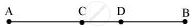# If a point C lies between two points A and B such that AC = BC, point C is called a mid-point of line segment AB. Prove that every line segment has one and only one mid-point. - Mathematics

If a point C lies between two points A and B such that AC = BC, point C is called a mid-point of line segment AB. Prove that every line segment has one and only one mid-point.

#### Solution

Let there be two mid-points, C and D.C is the mid-point of AB.

AC = CB

AC + AC = BC + AC        (Equals are added on both sides) … (1)

Here, (BC + AC) coincides with AB. It is known that things which coincide with one another are equal to one another.

∴ BC + AC = AB … (2)

It is also known that things which are equal to the same thing are equal to one another. Therefore, from equations (1) and (2), we obtain

AC + AC = AB

⇒ 2AC = AB … (3)

Similarly, by taking D as the mid-point of AB, it can be proved that

From equation (3) and (4), we obtain

2AC = 2AD (Things which are equal to the same thing are equal to one another.)

⇒ AC = AD (Things which are double of the same things are equal to one another.)

This is possible only when point C and D are representing a single point.

Hence, our assumption is wrong and there can be only one mid-point of a given line segment.

Concept: Euclid’S Definitions, Axioms and Postulates
Is there an error in this question or solution?

#### APPEARS IN

NCERT Class 9 Maths
Chapter 5 Introduction to Euclid's Geometry
Exercise 5.1 | Q 5 | Page 86